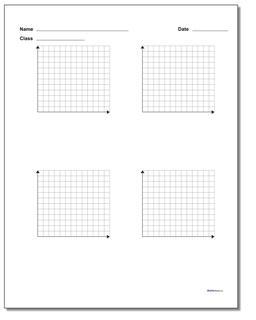# Math Worksheets: Coordinate Plane: Coordinate Plane: Four Problem Quadrant 1 Worksheet Paper (Second Worksheet)## Four Problem Quadrant 1 Worksheet Paper (Second Template)

PropertyValue
DescriptionFour Problem Quadrant 1 Worksheet Paper: This printable coordinate plane with quadrant 1 only shown is great for introducing graphing equations in 5th grade. (Second Template)
Resource TypeTemplate